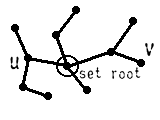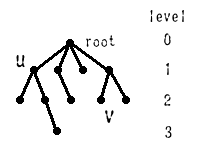Tree : undirected, connected, cycless graph

←→ undirected, there exists 1 path between any nodes u,v

• Rooted ( oriented ) treeFree tree

• Root

Parent, children, sibling

• Terminal node = leaf ( external )

Nonterminal node      ( internal )

• LevelHeight

Internal path length, I= 0 + 1 + 1 + 1 + 2

External path length, E= 2 + 3 + 2 + 1 + 2 + 2

• Binary tree : 0 or 2 children
• Property : N nodes trees → N-1 edges
• Property : Binary trees with N internal nodes

→ (1) N + 1 external nodes(2) E = I + 2N

proof : (1) external ←→ player

internal ←→ game

(2) E = I + ( #edges )

2 → E

1 →  I